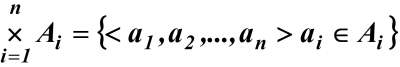# Sets and Symbols

## Introduction to Sets

• A Set is said to contain elements.
• Universal set U represents a collection of all objects within a particular context in which all other sets belong to U. For example all devices connected to the Internet.
• Set Notation: Roster form and Set Builder form
• In a Roster form, all the elements of a set are listed and separated by commas and enclosed between braces. For example S = {1,2,3}.
• An example of a set represented in a Builder form is the set S{e:e<0}, which is a set of all e such as e is less than 0.

## Common Universal Sets

• N = natural numbers = {0,1,2,...}
• Z = integer numbers = {...,-2,-1,0,1,2,...}
• Z+is the set of all positive integers
• R = real numbers including rational numbers such as the integers, fraction 5/4, and the irrational numbers such as square root of 3

### Other Notations

e is an element of S is written expressed as: e∈S. e is not an elements of S is written as: e∉S.

## Subsets

• Definition: The set A is a subset of the set B, denoted A⊆B, if and only if (iff) ∀x [x∈A → x∈B]
• Definition: The void set, the null set, the empty set, denoted ∅.
• Examples
• The assertion x∈ ∅ is always false.
• Therefore ∀x [x∈ ∅ → x ∈B] is always true. Hence, ∅ is a subset of every set.
• Any set is a subset of itself.
• Definition: If A ⊆B but A≠B is equivalent to say A is a proper subset of B, denoted A⊂B
• Definition: The set of all subsets of a set S is called the power set of S, denoted P(S)
• Example: If S = {x,y} then P(S) = {∅, {x}, {y}, {x,y}}
• Definition: The number of (distinct) elements in S, denoted▕S▏, is called the cardinality of S.
• The cardinality is a natural number (in N), then the set is called finite, else infinite.
• Examples
• S = {x,y},
• ▕{x,y}▏= 2
• ▕P({x,y})▏= 4
• S is finite and so is P(S)
• Hence, if ▕S▏ = n then ▕P(S)▏=2n
• Definition: The Cartesian product of A with B, denoted A×B, is the set of ordered pairs {(a, b) ▏a∈A∧b∈B} Notation:Example:
P = {a,b}, Q = {1, 2, 3}
P×Q = {(a, 1), (a, 2), (a, 3), (b, 1), (b, 2), (b, 3)}
Try to find to the following excercises:
Q×P and Q×Q
if ▕P▏ = l and ▕Q▏ = m then find ▕P×Q▏

## Set Operations

Definition: Two sets P and Q are equal, denoted P = Q, iff
∀x [x∈P↔x∈Q]
The symbol ↔ means logically equivalent (and also the statement is true if both parts are true, or both are false).
It is also important to note that the previous statement is also the same as:
P = Q iff ∀x [(x∈P→x∈Q) ∧ (x∈Q → x∈Q)]
P = Q iff P ⊆ Q and Q ⊆ P

### Intersection, Union, and Complement

Definitions:
• The union of P and Q, denoted P⋃Q, is the set {x ▏ x ∈P ⋁ x∈Q}
We can read this statement as follows: is the set where each element x such as x belongs to P or x belongs to Q.
• The intersection of P and Q, denoted P⋂Q, is the set where each of its elements belong to both sets P and Q, and it's expressed as follows:
{x ▏ x ∈P ⋀ x∈Q}
• The complement of P is denoted by P̄ is the set {x ▏ x ∉P}
• The difference of P and Q, or the complement of Q relative to P, denoted as P - Q, is the set P⋂Q̄
• The absolute complement of P is U - P
• Examples
U = {0, 1, 2, 3, 4, 5, 6, 7, 8, 9, 10}
P= {1, 2, 3, 4, 5}, Q = {4, 5, 6, 7, 8} Then
P⋃Q = {1, 2, 3, 4, 5, 6, 7, 8}
P⋂Q = {4, 5}
P̄= {0, 6, 7, 8, 9, 10}
Q̄= {0, 1, 2, 3, 9, 10}
P - Q= {1, 2, 3}
Q - P = {6, 7, 8}